Main Content

# dsp.SampleRateConverter

Multistage sample rate converter

## Description

The SampleRateConverter System object™ converts the sample rate of an incoming signal.

To convert the sample rate of a signal:

1. Create the dsp.SampleRateConverter object and set its properties.

2. Call the object with arguments, as if it were a function.

To learn more about how System objects work, see What Are System Objects?.

## Creation

### Syntax

src = dsp.SampleRateConverter
src = dsp.SampleRateConverter(Name,Value)

### Description

src = dsp.SampleRateConverter creates a multistage FIR sample rate converter System object, src, that converts the sample rate of each channel of an input signal.

example

src = dsp.SampleRateConverter(Name,Value) returns a multistage FIR sample rate converter System object, src, with properties and options specified by one or more Name,Value pair arguments.

## Properties

expand all

Unless otherwise indicated, properties are nontunable, which means you cannot change their values after calling the object. Objects lock when you call them, and the release function unlocks them.

If a property is tunable, you can change its value at any time.

For more information on changing property values, see System Design in MATLAB Using System Objects.

Specify the two-sided bandwidth of interest (after rate conversion) as a positive scalar expressed in Hz. This property sets the two-sided bandwidth of the information-carrying portion of the signal that you wish to retain.

Specify the sample rate of the input signal as a positive scalar expressed in Hz. The input sample rate must be greater than the bandwidth of interest.

Specify the maximum allowed tolerance for the sample rate of the output signal as a positive scalar between 0 and 1.

The output rate tolerance allows for a simpler design in many cases. The actual output sample rate varies but is within the specified range. For example, if OutputRateTolerance is specified as 0.01, then the actual output sample rate is in the range given by OutputSampleRate ± 1%.

Specify the sample rate of the output signal as a positive scalar expressed in Hz. The output sample rate must be greater than the bandwidth of interest.

Specify the stopband attenuation as a positive scalar expressed in dB. This property is the minimum amount by which any aliasing involved in the process is attenuated.

## Usage

### Syntax

y = src(x)

### Description

example

y = src(x) designs one or more multirate FIR filters and then uses the filters to convert the rate of each channel (column) of the real or complex input signal x to the output sampling rate.

### Input Arguments

expand all

Input signal, specified as a vector or a matrix. The row length of x must be a multiple of the overall decimation factor. The decimation factor is determined from the getRateChangeFactors function. Each column of x is treated as a separate channel.

Data Types: single | double
Complex Number Support: Yes

### Output Arguments

expand all

Resampled signal, returned as a vector or matrix.

Data Types: single | double
Complex Number Support: Yes

## Object Functions

To use an object function, specify the System object as the first input argument. For example, to release system resources of a System object named obj, use this syntax:

release(obj)

expand all

 getActualOutputRate Get actual output rate getFilters Obtain single-stage filters getRateChangeFactors Get overall interpolation and decimation factors visualizeFilterStages Visualize filter stages
 cost Implementation cost of the sample rate converter freqz Frequency response of the multirate multistage filter info Display information about sample rate converter
 step Run System object algorithm release Release resources and allow changes to System object property values and input characteristics reset Reset internal states of System object

## Examples

collapse all

Convert the sample rate of an audio signal from 44.1 kHz (CD quality) to 96 kHz (DVD quality).

Note: If you are using R2016a or an earlier release, replace each call to the object with the equivalent step syntax. For example, obj(x) becomes step(obj,x).

Note: The dsp.AudioFileWriter System object™ is not supported in MATLAB Online.

fs1 = 44.1e3; fs2 = 96e3; SRC = dsp.SampleRateConverter('Bandwidth',40e3,... 'InputSampleRate',fs1,'OutputSampleRate',fs2); [L,M] = getRateChangeFactors(SRC); FrameSize = 10*M; AR = dsp.AudioFileReader('guitar10min.ogg', ... 'SamplesPerFrame',FrameSize); AW = dsp.AudioFileWriter('guitar10min_96k.wav', ... 'SampleRate',fs2);

Run the system for 15 s. Release all objects.

tic while toc < 15 x = AR(); y = SRC(x); AW(y); end release(AR); release(AW); release(SRC);

Plot the input and output signals. Use a different set of axes for each signal. Shift the output to compensate for the delay introduced by the filter.

t1 = 0:1/fs1:1/30-1/fs1; t2 = 0:1/fs2:1/30-1/fs2; delay = 114; el1 = 1:length(t1)-delay; el2 = 1:length(t2); el2(1:delay) = []; subplot(2,1,1) plot(t1(1:length(el1)),x(el1,1)) hold on plot(t1(1:length(el1)),x(el1,2)) title('Input') subplot(2,1,2) plot(t2(1:length(el2)),y(el2,1)) hold on plot(t2(1:length(el2)),y(el2,2)) xlabel('Time (s)') title('Output')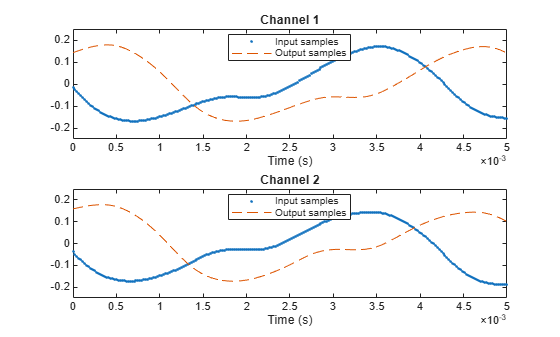Zoom in to see the difference in sample rates. Use a different set of axes for each channel.

figure subplot(2,1,1) plot(t1(1:length(el1)),x(el1,1),'o-') hold on plot(t2(1:length(el2)),y(el2,1),'d--') xlim([0.01 0.0103]) title('First channel') subplot(2,1,2) plot(t1(1:length(el1)),x(el1,2),'o-') hold on plot(t2(1:length(el2)),y(el2,2),'d--') xlim([0.01 0.0103]) xlabel('Time (s)') title('Second channel')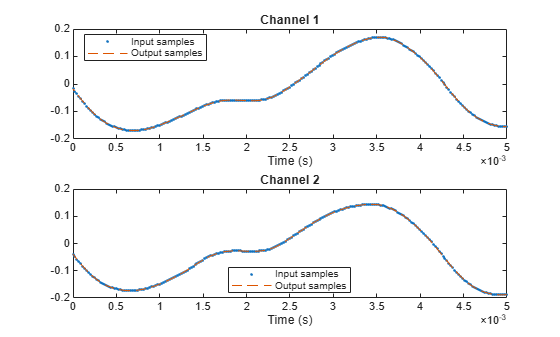Note: If you are using R2016a or an earlier release, replace each call to the object with the equivalent step syntax. For example, obj(x) becomes step(obj,x).

Create a multistage sample rate converter with default properties. The converter converts from 192 kHz to 44.1 kHz in three stages.

src = dsp.SampleRateConverter; 

Use src to convert the sample rate of a noisy sinusoid. The sinusoid has a frequency of 20 kHz and is sampled for 0.1 s.

f = 20e3; FsIn = src.InputSampleRate; FsOut = src.OutputSampleRate; t1 = (0:1/FsIn:0.1-1/FsIn)'; sIn = sin(2*pi*f*t1) + randn(size(t1)); 

Estimate the power spectral density of the input.

hsa = dsp.SpectrumAnalyzer('SampleRate',FsIn,'YLimits',[-40 40]); hsa(sIn)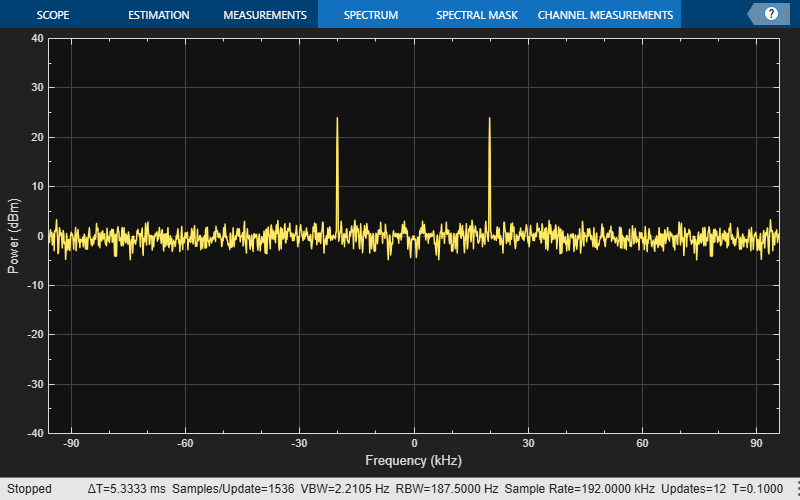Convert the sample rate of the signal. Estimate the power spectral density of the output.

sOut = src(sIn); hsb = dsp.SpectrumAnalyzer('SampleRate',FsOut,'YLimits',[-40 40]); hsb(sOut)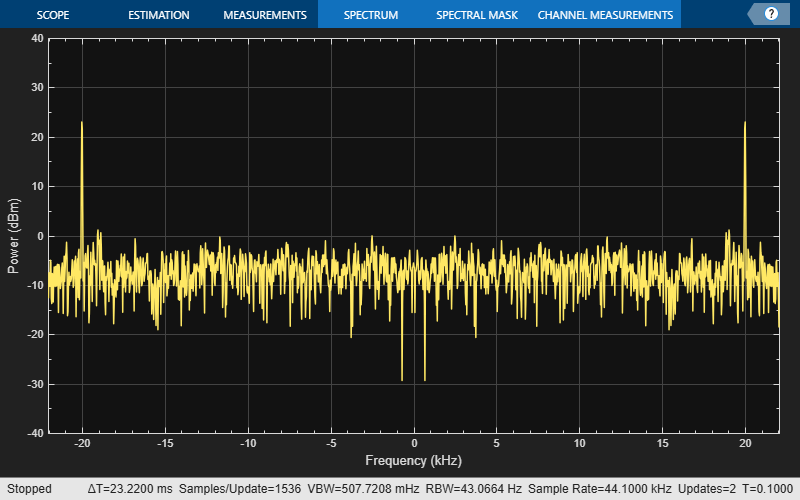A signal output from an A/D converter is sampled at 98.304 MHz. The signal has a bandwidth of 20 MHz. Reduce the sample rate of the signal to 22 MHz, which is the bandwidth of 802.11 channels. Make the conversion exactly and then redo it with an output rate tolerance of 1%.

SRC1 = dsp.SampleRateConverter('Bandwidth',20e6, ... 'InputSampleRate',98.304e6,'OutputSampleRate',22e6, ... 'OutputRateTolerance',0); SRC2 = dsp.SampleRateConverter('Bandwidth',20e6, ... 'InputSampleRate',98.304e6,'OutputSampleRate',22e6, ... 'OutputRateTolerance',0.01);

Use the cost method to determine the cost of each sample rate conversion. The zero-tolerance process requires more than 500 times as many coefficients as the 1% process.

c1 = cost(SRC1)
c1 = struct with fields: NumCoefficients: 84779 NumStates: 133 MultiplicationsPerInputSample: 27.0422 AdditionsPerInputSample: 26.0684 
c2 = cost(SRC2)
c2 = struct with fields: NumCoefficients: 150 NumStates: 127 MultiplicationsPerInputSample: 22.6667 AdditionsPerInputSample: 22.1111 

Find the integer upsampling and downsampling factors used in each conversion.

[L1,M1] = getRateChangeFactors(SRC1)
L1 = 1375 
M1 = 6144 
[L2,M2] = getRateChangeFactors(SRC2)
L2 = 2 
M2 = 9 

Compute the actual sample rate of the output signal when the sample rate conversion has a tolerance of 1%.

getActualOutputRate(SRC2)
ans = 2.1845e+07 

Note: If you are using R2016a or an earlier release, replace each call to the object with the equivalent step syntax. For example, obj(x) becomes step(obj,x).

Create a multistage sample rate converter with default properties, corresponding to the combined three filter stages used to convert from 192 kHz to 44.1 kHz. Determine its overall decimation and interpolation factors.

src = dsp.SampleRateConverter; [L,M] = getRateChangeFactors(src);

Create a two-channel random signal. Specify a number of samples equal to the decimation factor. Call the object twice on the signal.

x = randn(M,2); y1 = src(x); y2 = src(x); no = all(y2==y1)
no = 1x2 logical array 0 0 

The output is different because the internal states of src have changed. Use reset to reset the converter and call the converter again. Verify that the output is unchanged.

reset(src) y3 = src(x); yes = all(y3==y1)
yes = 1x2 logical array 1 1 

Create a multistage sample rate converter with default properties, corresponding to the combined three filter stages used to convert from 192 kHz to 44.1 kHz. Compute and display the frequency response.

src = dsp.SampleRateConverter; [H,f] = freqz(src); plot(f,20*log10(abs(H)))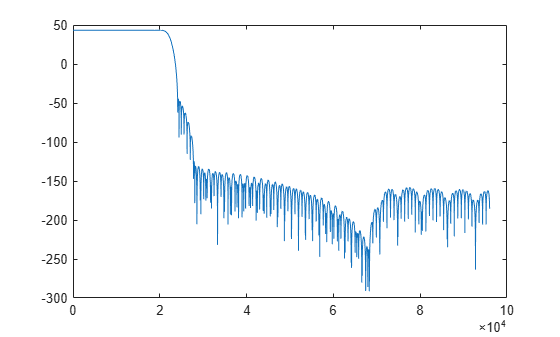Compute and display the frequency response over the range between 20 Hz and 44.1 kHz.

f = 20:10:44.1e3; [H,f] = freqz(src,f); plot(f,20*log10(abs(H)))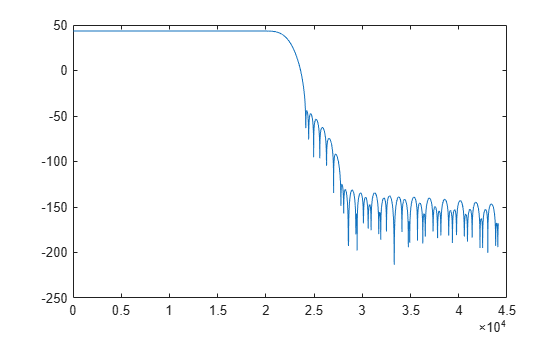Create src, a multistage sample rate converter with default properties. src converts between 192 kHz and 44.1 kHz. Find the individual filters that are cascaded together to perform the conversion.

src = dsp.SampleRateConverter; c = getFilters(src);

Visualize the frequency response of the decimator used in the first stage of the process.

m = c.Stage1; [h,w] = freqz(m); plot(w/pi,20*log10(abs(h))) xlabel('\omega / \pi') ylabel('Magnitude (dB)')Create a multistage sample rate converter with default properties, corresponding to the combined three filter stages used to convert from 192 kHz to 44.1 kHz.

src = dsp.SampleRateConverter
src = dsp.SampleRateConverter with properties: InputSampleRate: 192000 OutputSampleRate: 44100 OutputRateTolerance: 0 Bandwidth: 40000 StopbandAttenuation: 80 

Display information about the design.

info(src)
ans = 'Overall Interpolation Factor : 147 Overall Decimation Factor : 640 Number of Filters : 3 Multiplications per Input Sample: 27.667188 Number of Coefficients : 8631 Filters: Filter 1: dsp.FIRDecimator - Decimation Factor : 2 Filter 2: dsp.FIRDecimator - Decimation Factor : 2 Filter 3: dsp.FIRRateConverter - Interpolation Factor: 147 - Decimation Factor : 160 ' 

Get the actual output sample rate for conversion between 192 kHz and 44.1 kHz when given a tolerance of 1%.

src = dsp.SampleRateConverter; src.OutputRateTolerance = 0.01; FsOut = getActualOutputRate(src)
FsOut = 4.4308e+04 

Create src, a multistage sample rate converter with default properties. src combines three filter stages to convert from 192 kHz to 44.1 kHz. Determine its overall interpolation and decimation factors.

src = dsp.SampleRateConverter; [L,M] = getRateChangeFactors(src)
L = 147 
M = 640 

Create a multistage sample rate converter with default properties, corresponding to the combined three filter stages used to convert from 192 kHz to 44.1 kHz. Visualize the stages.

src = dsp.SampleRateConverter; visualizeFilterStages(src)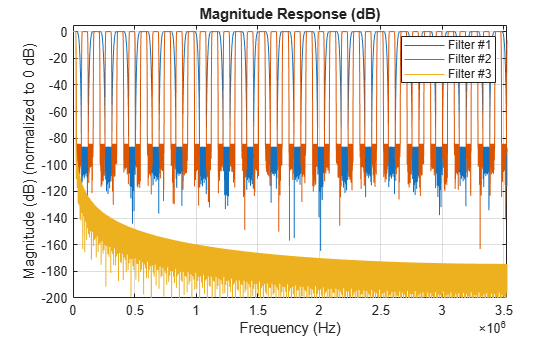## Algorithms

• The general multistage sample rate converter performs a multistage decimation, a single-stage sample rate conversion, and a multistage interpolation, in that order. Actual designs include at most two of those steps.

• The procedure determines automatically the optimal number of decimation or interpolation stages. In special cases, the decimation or the interpolation can be performed in a single stage.

• The algorithm always attempts to start by reducing the sample rate. This decreases the amount of computation required. The decimation step is designed so that no intermediate sample rate goes below the bandwidth of interest. This ensures that no information is filtered out.

• Each individual stage uses halfband or Nyquist filters to minimize the number of nonzero coefficients.

• Transition-band aliasing is allowed because it decreases the implementation cost. The signal within the bandwidth of interest is kept alias free up to the value specified by the StopbandAttenuation property.

## Extended Capabilities

Introduced in R2014b

## SupportGet trial now